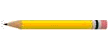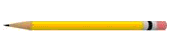# Length

Length can be measured in metric units or in U.S. customary units .

## Metric Units

The standard metric unit of length is the meter , which is a bit longer than $3$ feet.

(The exact length of a meter is defined by the International Bureau of Weights and Measures as the length of the path travelled by light in vacuum during a very short interval of time, namely $\frac{1}{299972458}$ of a second. Don't worry, you will probably never have to remember this!)

From the meter, we get the rest of the metric units using the standard metric prefixes.

 nanometer(nm) $\frac{1}{1,000,000,000}$ of a meter micrometer(um) $\frac{1}{1,000,000}$ of a meter millimeter(mm) $\frac{1}{1000}$ of a meter centimeter(cm) $\frac{1}{100}$ of a meter decimeter(dm) $\frac{1}{10}$ of a meter meter(m) $1$ meter dekameter(dam) $10$ meters hectometer(hm) $100$ meters kilometer(km) $1000$ meters Megameter(Mm) $1,000,000$ meters Gigameter(Gm) $1,000,000,000$ meters Terameter(Tm) $1,000,000,000,000$ meters

Metric rulers are usually have centimeters numbered, with smaller marks shown for millimeters.The pencil in the figure has a length of $3.4$ centimeters, or $34$ millimeters.

## U.S. Customary Units

The system for measuring length in the customary system is based on the inch, foot, yard, and mile.

$\overline{)\begin{array}{|l|}\hline 1\text{\hspace{0.17em}}\text{\hspace{0.17em}}\text{foot}\text{\hspace{0.17em}}\left(\text{ft}\right)\text{\hspace{0.17em}}\text{\hspace{0.17em}}\text{\hspace{0.17em}}\text{\hspace{0.17em}}\text{\hspace{0.17em}}\text{\hspace{0.17em}}=\text{\hspace{0.17em}}\text{\hspace{0.17em}}12\text{\hspace{0.17em}}\text{inches}\text{\hspace{0.17em}}\text{\hspace{0.17em}}\left(\text{in}.\right)\\ 1\text{\hspace{0.17em}}\text{\hspace{0.17em}}\text{yard}\text{\hspace{0.17em}}\text{\hspace{0.17em}}\left(\text{yd}\right)\text{\hspace{0.17em}}\text{\hspace{0.17em}}=\text{\hspace{0.17em}}\text{\hspace{0.17em}}3\text{\hspace{0.17em}}\text{feet}\\ \text{1}\text{\hspace{0.17em}}\text{\hspace{0.17em}}\text{mile}\text{\hspace{0.17em}}\text{\hspace{0.17em}}\text{(mi)}\text{\hspace{0.17em}}\text{\hspace{0.17em}}\text{\hspace{0.17em}}\text{=}\text{\hspace{0.17em}}\text{\hspace{0.17em}}\text{5,280}\text{\hspace{0.17em}}\text{\hspace{0.17em}}\text{feet}\\ \hline\end{array}}$

Lengths smaller than $1$ inch are usually written using fractions of an inch. On a U.S. customary foot ruler, the inch is usually divided into halves, quarters, eighths, and sixteenths by progressively smaller markings.In the figure shown, the pencil is $2\frac{5}{16}$ inches long.

To convert from one unit to another, we can use unit analysis . For instance, if you want to find the number of inches in $3$ miles, you can multiply the quantity $3$ miles by the fraction $5280\text{\hspace{0.17em}}\text{\hspace{0.17em}}\text{feet}/1$ mile and then by the fraction $12\text{\hspace{0.17em}}\text{\hspace{0.17em}}\text{inches}/1$ foot.

$\begin{array}{l}1\text{\hspace{0.17em}}\text{\hspace{0.17em}}\text{mile}=5,280\text{\hspace{0.17em}}\text{\hspace{0.17em}}\text{feet}\\ 1\text{\hspace{0.17em}}\text{\hspace{0.17em}}\text{foot}=12\text{\hspace{0.17em}}\text{\hspace{0.17em}}\text{inches}\end{array}$

$12\text{\hspace{0.17em}}\text{\hspace{0.17em}}\text{inches}\text{\hspace{0.17em}}\text{\hspace{0.17em}}×\text{\hspace{0.17em}}\text{\hspace{0.17em}}5,280\text{\hspace{0.17em}}\text{\hspace{0.17em}}\text{feet}=63,360$ inches in $1$ mile

To find the number of inches in $3$ miles:

$\frac{3×5280\text{\hspace{0.17em}}\text{\hspace{0.17em}}\overline{)\text{feet}}}{1\text{\hspace{0.17em}}\text{\hspace{0.17em}}\text{mile}}×\frac{12}{1\text{\hspace{0.17em}}\text{\hspace{0.17em}}\overline{)\text{foot}}}=190080\text{\hspace{0.17em}}\text{\hspace{0.17em}}\text{inches}$

(Both these fractions are equal to $1$ , so you're not changing the original quantity when you multiply.)

Note:

(a) To convert from larger units to smaller units, multiply by the appropriate unit ratio.

(b) To convert from smaller units to larger units, multiply by the reciprocal of the appropriate unit ratio.

(c) Multiplying or dividing by a unit ratio is mathematically equivalent to using a proportion to convert between units of measure .